Next: FASCOD3P Model Up: Infrared Spectrum Previous: Infrared Spectrum

Given a temperature profile and vertical distribution of gaseous constituents in a clear atmosphere, one can derive the radiance if the spectroscopic properties of the gas are also known. For monochromatic radiation, the differential transmissivity through the atmosphere is determined by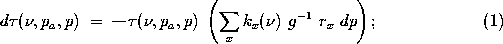where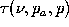= atmospheric transmission from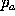to p;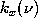= absorption of atmospheric constituent x, mkg;= wavenumber, cm; g = gravitational acceleration, m s;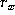= mixing ratio of constituent x, g kg; and p = pressure at given level, hPa.

Atmospheric pressure units are often expressed in mb, and are equivalent to hPa.

The general radiative transfer equation (RTE) for downwelling spectral radiance in a clear atmosphere is described by the relation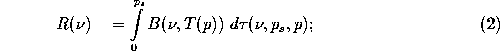where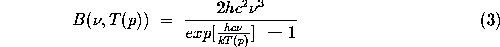represents the Planck radiance, mW (msr cm);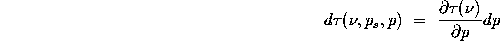is the differential transmission over the pressure layers;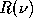= AERI measured downwelling column radiance, mW (msr cm); T(p) = temperature at pressure level p, K; h = Planck's constant, J s; c = speed of light, m s; k = Boltzmann's constant, J K;

and the integral limits,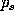and 0, specify surface layer and top of atmosphere, respectively.

The radiance is often described in terms of a temperature because it removes the spectral dependence, normalizing the data with respect to the Planck curve. This `effective temperature' is referred to as the brightness temperature,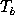, and is the solution of Equation 3 for T(p), in terms of the measured radiance,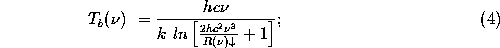where the measured downwelling column radiance,, has been substituted for the Planck radiance,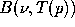.

Equation 4 will yield small errors when used for a spectral bandpass rather than a single wavenumber; where the bandpass would be represented by the mean wavenumber,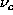. A correction, based on a least-squares fit of the measured radiance in a given bandpass over a typical temperature domain, can be applied to Equation 4, such that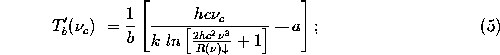where a and b are the least-squares fit y-intercept and slope, respectively. Appendix B summarizes this approach and illustrates the associated errors.

Additional absorption and radiative feedback must be accounted for if a cloud is introduced to the atmosphere. When this occurs, the atmosphere can be partitioned into layers: clear sky below the cloud, cloud layer, and clear sky above the cloud; assuming a single cloud layer. Equation 2, the clear sky RTE, would be adjusted as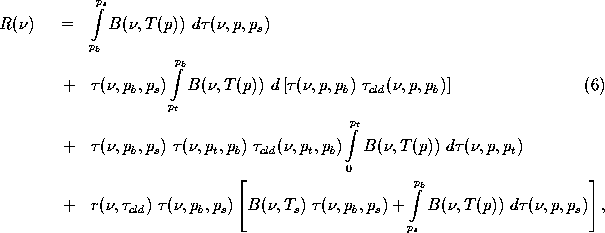where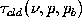= cloud transmissivity, from cloud base to p;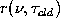= angular integrated cloud reflectivity;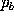= cloud base pressure, hPa; and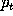= cloud top pressure, hPa.

The first three terms of Equation 6 are an expansion of Equation 2, whereas the last term accounts for the reflection of upwelling terrestrial and atmospheric radiation from below the cloud. A cloud particle size distribution outside the 50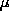m radius reflectance parameterization yields a very small (see Appendix D) change in the radiance. This accounts for the approximation in Equation 6.

The following sections discuss the individual terms of the cloudy RTE, where: an atmospheric transmission model is used to calculate the clear sky values; an evaluation of cloud optical properties will lead to a cloud reflectivity term; and the RTE can be inverted to derive an optical depth for the cloud. The final section describes a technique to determine an estimate of the optical depth using the 9.6m ozone band.Next: FASCOD3P Model Up: Infrared Spectrum Previous: Infrared Spectrum

Daniel DeSlover
Sun Aug 11 10:02:40 CDT 1996